Courses

# Test: Percentages- 3

## 15 Questions MCQ Test General Aptitude for GATE 2020 | Test: Percentages- 3

Description
This mock test of Test: Percentages- 3 for Quant helps you for every Quant entrance exam. This contains 15 Multiple Choice Questions for Quant Test: Percentages- 3 (mcq) to study with solutions a complete question bank. The solved questions answers in this Test: Percentages- 3 quiz give you a good mix of easy questions and tough questions. Quant students definitely take this Test: Percentages- 3 exercise for a better result in the exam. You can find other Test: Percentages- 3 extra questions, long questions & short questions for Quant on EduRev as well by searching above.
QUESTION: 1

### What fraction is equal to 57.12% (approximately)?

Solution: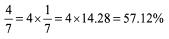QUESTION: 2

### a% of a + b% of b = 2% of ab, then what percentage of a is b?

Solution: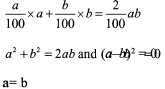QUESTION: 3

### Subtracting 48% of N from N is equivalent to multiplying N by

Solution:

N - 0.48 N = 0.52 N

QUESTION: 4

The length of rectangle is increased by 30% and the breadth is decreased by 25%. What is the percentage change in the area of the rectangle due to this?

Solution:

Let Length = 100, Breadth =100 Hence, Area = 10000

Now, Length =130, Breadth = 75 Hence, Area = 130 x 75 = 9750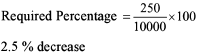QUESTION: 5

The numbers are respectively 30% and 40% of a third number. What percentage is the first number of the second number?

Solution:

Let 3rd No = 100

1st No. = 30 and 2nd No. = 40 Hence, 1st is 75% of 2nd No.

QUESTION: 6

A man spends 25% of his money on food. After spending 50% of the remaining, he is left with Rs 375. How much money was with that man initially?

Solution:

Assume that he had initially Rs 100.

After spending on food, money left = Rs 75

After spending 50% of Rs 75, he had Rs 37.5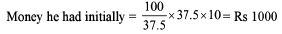QUESTION: 7

After spending 20% on clothes, 10% on books, 9% on purchasing gift for girl friend and 7% on others, Chandra has a balance of Rs 2,700. How much money was there with him initially?

Solution:

Let initial Amount = Rs. 100

Money left = 100 – (20 + 10 + 9 + 7)

= Rs. (100 – 46)

= Rs. 54

This 54r = 2700

r = 50

100r = 5000

QUESTION: 8

In an exam of two papers maths and chemistry, 60% of the students pass in maths and 70% pass in chemistry. What is minimum percentage of students who could have failed in both the subjects?

Solution:

Since, we have to find the minimum percentage, it can be possible that no one has failed in both the subjects.

QUESTION: 9

An amount is lent at x% p.a. simple interest for two years. Instead, had it been lent at 2x% p.a. simple interest for y more years, then the interest would have been five times the earlier interest. Find the value of y.

Solution: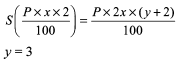QUESTION: 10

Find the least number of integral years in which a sum of money invested at 20% compound interest per annum will become more than double itself.

Solution: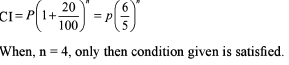QUESTION: 11

In the country of Tattoland, a new term tercentage has been introduced in the place of percentage. While percentage is per 100, tercentage is per 125. What will be the tercentage marks obtained by a student in Tattoland if his percentage of marks is 60?

Solution:

The numerator has been increased by 25%. Hence, denominator will also increase by 25%.
Value in tercentage = 60 x 1.25 = 75 tercentage

QUESTION: 12

In a maternity centre, 5% of all the childbirth cases result in twins. What is the approximate percentage of twins out of total children born?

Solution:

Let there be x number of birth cases.
Thus, the number of birth cases of twins = (5*x)/100 = x/20
So the total number of children born are = x + (x/20)(since in cases of twins there is an additional child) = 21x/20
Total number of twin born = (x/20)* 2 = x/10
percentage of twins in the total population born = ((x/10)/(21x/20)) * 100 = 9.5 %

QUESTION: 13

Three friends P, Q and R donate 8%, 7% and 9% of their salary to a charitable hospital in the given order. Salary of P and Q is same and the difference of their donations is Rs 74. The total donation by the P and Q is Rs 525 more than R’s donation. What is the percentage of the total salary of the three donations ?

Solution: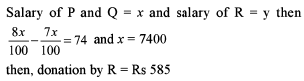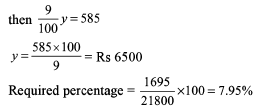QUESTION: 14

In solution of sugar and water the ratio of sugar and water by weight is 1:4. This solution is heated and in the process it loses 50% weight. What is the ratio of weight of sugar and water in the final mixture?

Solution:

Weight of solution = (1+4) = 5 units weight of sugar = 1 unit weight of water after heating =1.5 units Required ration =1 : 1.5 = 2 : 3

QUESTION: 15

Dataman Infosys Systems is a Lucknow-based software company which is growing tremendously. It doubled its turnover in 1998 from Rs 50 crores in 1997. Then it tripled its turnover in 1999 and grew by 50% in 2000. What is the turnover at the end of 2000?

Solution:

Turnover in 1998 = Rs 100 cr Turnover in 1999 = Rs 300 cr Turnover in 2000 = Rs 450 cr